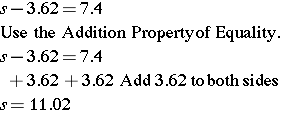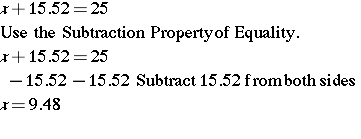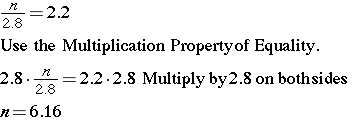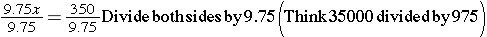# Solve Equations with Decimals

In this lesson, you will learn to solve equations containing decimals. Let us begin with an activity involving mental math and estimation.

In the following mathematical sentences, use mental math to find the missing number. Write your answers down.

1. 14.75 + _____ = 30

2. 10 – _____ = 5.5

3. 3 * _____ = 7.5

4. _____ ÷ 0.5 = 6

In the following equations, use a calculator to find the value of x.

5. 14.75 + x = 30

6. 10 – x = 5.5

7. 3 * x = 7.5

8. x ÷ 0.5 = 6

Notice that the answers in both sections are the same.

## Investigate

Students on the cross-country team were running 50-yard dashes as part of a fitness test. The slowest time on the team was 3.62 seconds slower than the fastest time of 7.4 seconds. You can write an equation to represent this situation. The slowest time s minus 3.62 is equal to the fastest time of 7.4 seconds.

• Slowest time minus 3.62 is 7.4.
• S – 3.62 = 7.4

### Example 1

Solve equations by adding or subtracting.### Example 2

Solve equations by multiplying or dividing.## InvestigateKayce wants to purchase a new wakeboard that costs \$350.00. If she earns \$9.75 per hour at work, how many hours must she work to earn enough money to buy the wakeboard?

Strategy

First, rewrite the question as a statement.

Find the number of hours Kayce must work to earn the \$350.00. Translate the words into math. Use a variable to represent the number of hours.Solution## Practice

1) -0.7 = -7.8 + a
2) 9.1 + b = 7.7
3) -1.6 = x – 8.7
4) 10.1 = p + 3.8
5) r + 0.2 = -7.8
6) n + 1.3 = 7.9
7) -8.5x = 58.65
8) x/7.5 = 0.24
9) 2.5 + a = 12

(source)